# Python Logical Operators

A logical operator is the one that compares one or more values and return Boolean true or false. Python has a set of logical operators that are used in conditionals and loops. The python programming language supports following logical operators:

1. Relational operators
2. Set membership operators
3. Boolean operators

### Relational Operators

Lets us list all the logical operators.

The above is the list of python relational operators. A relational operator in python compares both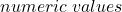and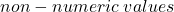and return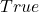or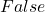. Let us see few examples.

In the following examples, we will check equality of two numbers and print the results.

# Checking equality of two numbers
print(10 == 10)
print(23 == 12)
print(45 != 56)
# Checking equality of two variable containing numbers
num1 = 200
num2 = 100
print(num1 == num2)

The program above will print following results.

= RESTART: C:/PROGRAMMING/PythonExercises/Relational Operators/Numeric-Equalilty.py
True
False
True
False
>>> 

The value of variable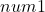does not match with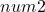, therefore, the output is. The same is tested for other equality. In python, you can also check the equality of a string which is a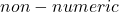value.

In the example below, we will check the equality of two strings.

str1 = "IT Employee"
str2 = "IT Employee"
str3 = "It Employee"
print(str1 == str2)
print(str2 == str3)

The python code above compares each character of both strings at corresponding location. It is going to be True, if

• The length of the strings match
• Each of the characters match at each position starting with 0.

Output of the above code is given below.

= RESTART: C:/PROGRAMMING/PythonExercises/Relational Operators/Non-Numeric_Quality.py
True
False
>>> 

In the case,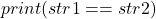, both string length and characters are equal, therefore, the program returns. In the second case,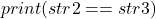is different by one character, therefore, program returns. Let us now check the relational operator for greater than or less that another number.

# Check if number is grater than another number
number1 = 35
number2 = 44
print(number1 < number2)
# Check if string is greater than another string
str1 = "Hello"
str2 = "hello"
print(str1 > str2)

In the above example,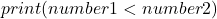is true because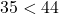. But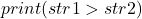is false. You need to understand why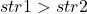is false. The different between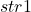and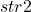is letter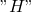and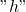respectively. In python, letters are in following sequence.

A \hspace{3px}B \hspace{3px}C\hspace{3px} D \hspace{3px}E\hspace{3px} F \hspace{3px}G \hspace{3px}... \hspace{3px}a \hspace{3px} b \hspace{3px}c \hspace{3px}d \hspace{3px}e...

Since,comes after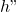, therefore,is greater than. Also, consider another capital aftersuch as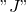. The letteris also greater thanbecause it comes after.

### Set Membership Operators

The set membership operators check if a number belong to a particular set. If it belongs then return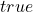, else return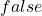. Here is the list of set membership operators.

In the example, we will check a list of fruits for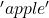and output our result.

my_list = ['oranges','grapes','apple','banana']
print('apple' in my_list)
print('mango' not in my_list)

The program checks forin the list and also, checks if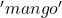is not in the list. The output of the program is as follows.

= RESTART: C:/PROGRAMMING/PythonExercises/Relational Operators/Set_Membership_operators.py
True
True
>>> 

The output is true for both statements involving set operators.

### Boolean Operators

The Boolean operators are for complex conditions, when there are multiple conditions that need to be checked before a block of code executes in python. Here is a list of Boolean operators.

Logical AND – compares two Boolean values and if both of them are true then return, otherwise, return. Here is an example.

exp = 3 > 2
exp2 = 5 > 3
if exp and exp2:
print("condition is true")
else:
print("condition is false")

The above program checks both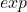and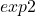. If both are true, only then it prints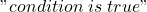. Otherwise, it will prints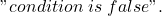. The output is given below.

>>>
== RESTART: C:/PROGRAMMING/PythonExercises/Relational Operators/Logical AND.py =
condition is true
>>> 

Logical OR – compares two Boolean values and if any one of them is, it returns a true. Otherwise,.

exp = 3 > 7
exp2 = 5 > 3
if exp or exp2:
print("condition is true")
else:
print("condition is false")

Theis false, but theis true which is enough to make the condition true and it prints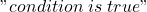as output. The output is given below.

== RESTART: C:/PROGRAMMING/PythonExercises/Relational Operators/logical OR.py ==
condition is true
>>> 

Truth table for the logical OR is given below.

### Logical NOT

This operator is also known as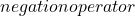. it will negate anystatement intoandstatement into. Consider the following example.

# The my_exp is false at the beginning
my_exp = 233 > 555
# Convert my_exp into true
if not(my_exp):
print("This statement is true now!")
print("Done execution")

The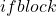will never execute unless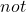operator convert the condition into. The output is given below.

== RESTART: C:/PROGRAMMING/PythonExercises/Relational Operators/logical NOT.py =
This statement is true now!
Done execution
>>> 

Let us look at the truth table for logical NOT.

Note: since, there is only one variable, the number of row is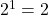, if variable is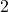, then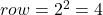.